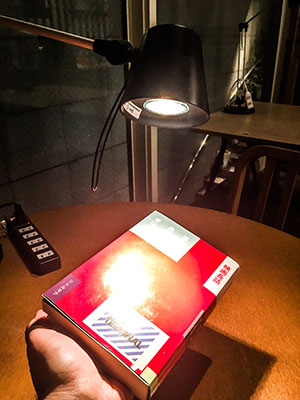# WebGLFundamentals.org

Fix, Fork, Contribute

# WebGL 三维点光源

``````uniform vec3 u_lightWorldPosition;
``````

``````uniform mat4 u_world;

...

// 计算表面的世界坐标
vec3 surfaceWorldPosition = (u_world * a_position).xyz;
``````

``````v_surfaceToLight = u_lightWorldPosition - surfaceWorldPosition;
``````

``````attribute vec4 a_position;
attribute vec3 a_normal;

+uniform vec3 u_lightWorldPosition;

+uniform mat4 u_world;
uniform mat4 u_worldViewProjection;
uniform mat4 u_worldInverseTranspose;

varying vec3 v_normal;

+varying vec3 v_surfaceToLight;

void main() {
// 将位置和矩阵相乘
gl_Position = u_worldViewProjection * a_position;

// 重定向法向量并传递给片段着色器
v_normal = mat3(u_worldInverseTranspose) * a_normal;

+  // 计算表面的世界坐标
+  vec3 surfaceWorldPosition = (u_world * a_position).xyz;
+
+  // 计算表面到光源的方向
+  // 传递给片段着色器
+  v_surfaceToLight = u_lightWorldPosition - surfaceWorldPosition;
}
``````

``````precision mediump float;

// 从顶点着色器中传入的值
varying vec3 v_normal;
+varying vec3 v_surfaceToLight;

-uniform vec3 u_reverseLightDirection;
uniform vec4 u_color;

void main() {
// 由于 v_normal 是可变量，所以经过插值后不再是单位向量，
// 单位化后会成为单位向量
vec3 normal = normalize(v_normal);

vec3 surfaceToLightDirection = normalize(v_surfaceToLight);

-float light = dot(normal, u_reverseLightDirection);
+float light = dot(normal, surfaceToLightDirection);

gl_FragColor = u_color;

// 只将颜色部分（不包含 alpha） 和光照相乘
gl_FragColor.rgb *= light;
}
``````

``````-  var reverseLightDirectionLocation =
-      gl.getUniformLocation(program, "u_reverseLightDirection");
+  var lightWorldPositionLocation =
+      gl.getUniformLocation(program, "u_lightWorldPosition");
+  var worldLocation =
+      gl.getUniformLocation(program, "u_world");
``````

``````  // 设置矩阵
+  gl.uniformMatrix4fv(
+      worldLocation, false,
+      worldMatrix);
gl.uniformMatrix4fv(
worldViewProjectionLocation, false,
worldViewProjectionMatrix);

...

-  // 设置光照方向
-  gl.uniform3fv(reverseLightDirectionLocation, m4.normalize([0.5, 0.7, 1]));
+  // 设置光源位置
+  gl.uniform3fv(lightWorldPositionLocation, [20, 30, 50]);
````````````attribute vec4 a_position;
attribute vec3 a_normal;

uniform vec3 u_lightWorldPosition;
+uniform vec3 u_viewWorldPosition;

uniform mat4 u_world;
uniform mat4 u_worldViewProjection;
uniform mat4 u_worldInverseTranspose;

varying vec3 v_normal;

varying vec3 v_surfaceToLight;
+varying vec3 v_surfaceToView;

void main() {
// 将位置和矩阵相乘
gl_Position = u_worldViewProjection * a_position;

// 重定向法向量并传递到片段着色器
v_normal = mat3(u_worldInverseTranspose) * a_normal;

// 计算表面的世界坐标
vec3 surfaceWorldPosition = (u_world * a_position).xyz;

// 计算表面到光源的方向
// 然后传递到片段着色器
v_surfaceToLight = u_lightWorldPosition - surfaceWorldPosition;

+  // 计算表面到相机的方向
+  // 然后传递到片段着色器
+  v_surfaceToView = u_viewWorldPosition - surfaceWorldPosition;
}
``````

``````// 从顶点着色器中传入的值
varying vec3 v_normal;
varying vec3 v_surfaceToLight;
+varying vec3 v_surfaceToView;

uniform vec4 u_color;

void main() {
// 由于 v_normal 是可变量，所以经过插值后不再是单位向量，
// 单位化后会成为单位向量
vec3 normal = normalize(v_normal);

+  vec3 surfaceToLightDirection = normalize(v_surfaceToLight);
+  vec3 surfaceToViewDirection = normalize(v_surfaceToView);
+  vec3 halfVector = normalize(surfaceToLightDirection + surfaceToViewDirection);

float light = dot(normal, surfaceToLightDirection);
+  float specular = dot(normal, halfVector);

gl_FragColor = u_color;

// 只将颜色部分（不包含 alpha） 和光照相乘
gl_FragColor.rgb *= light;

+  // 直接加上高光
+  gl_FragColor.rgb += specular;
}
``````

``````var lightWorldPositionLocation =
gl.getUniformLocation(program, "u_lightWorldPosition");
+var viewWorldPositionLocation =
+    gl.getUniformLocation(program, "u_viewWorldPosition");

...

// 计算相机矩阵
var camera = [100, 150, 200];
var target = [0, 35, 0];
var up = [0, 1, 0];
var cameraMatrix = makeLookAt(camera, target, up);

+// 设置相机位置
+gl.uniform3fv(viewWorldPositionLocation, camera);
``````

``````uniform vec4 u_color;
+uniform float u_shininess;

...

-  float specular = dot(normal, halfVector);
+  float specular = 0.0;
+  if (light > 0.0) {
+    specular = pow(dot(normal, halfVector), u_shininess);
+  }
``````

``````+var shininessLocation = gl.getUniformLocation(program, "u_shininess");

...

// 设置亮度
gl.uniform1f(shininessLocation, shininess);
``````

``````uniform vec4 u_color;
uniform float u_shininess;
+uniform vec3 u_lightColor;
+uniform vec3 u_specularColor;

...

// 只将颜色部分（不包含 alpha） 和光照相乘
*  gl_FragColor.rgb *= light * u_lightColor;

// 直接和高光相加
*  gl_FragColor.rgb += specular * u_specularColor;
}
``````

``````+  var lightColorLocation =
+      gl.getUniformLocation(program, "u_lightColor");
+  var specularColorLocation =
+      gl.getUniformLocation(program, "u_specularColor");
``````

``````// 设置光照颜色
+  gl.uniform3fv(lightColorLocation, m4.normalize([1, 0.6, 0.6]));  // 红光
// 设置高光颜色
+  gl.uniform3fv(specularColorLocation, m4.normalize([1, 0.6, 0.6]));  // 红光
``````

### 为什么 `pow(negative, power)` 是 undefined?

`pow(5, 2)`

`5 * 5 = 25`

`pow(5, 3)`

`5 * 5 * 5 = 125`

`pow(-5, 2)`

`-5 * -5 = 25`

`pow(-5, 3)`

`-5 * -5 * -5 = -125`

`pow(-5, 2.5)`

.
• 基础概念
• 图像处理
• 二维平移，旋转，缩放和矩阵运算
• 三维
• 光照
• 组织和重构
• 几何
• 纹理
• 渲染到纹理
• 阴影
• 技术
• 建议
• 优化
• 杂项
• 参考

Issue/Bug? 在GitHub上提issue.